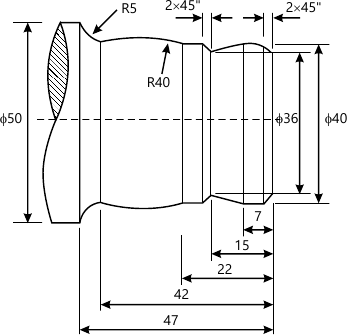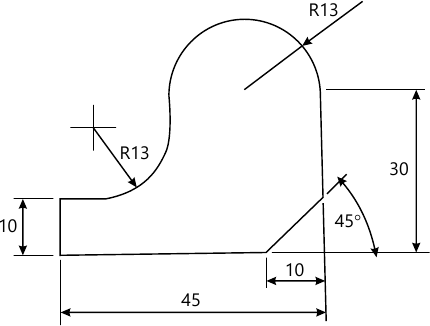MU Mechanical Engineering (Semester 7)
December 2014
Total marks: --
Total time: --
INSTRUCTIONS
(1) Assume appropriate data and state your reasons
(2) Marks are given to the right of every question
(3) Draw neat diagrams wherever necessary

1 (a) Write short note on significance of rapid prototyping in product development and testing.
6 M
1 (b) Write short note on automated material handling and storage systems.
7 M
1 (c) Find the equation of a Bezier curve which is defined by the four points as P0(2,2,0), P1(3,3,0) and P3(3,2,0) and also find the points on the curve for u=0, 0.25, 0.5, 0.75, and 1.
7 M

2 (a) Write a part program using G and M codes for finishing a forged component as shown in Figure 1. Assume the speed and feed on the turning centre as 500 RPM and 0.3 mm/rev respectively. Furthermore, assume 1mm material is to be removed radially from external diameter.10 M
2 (b) A triangle PQR has its vertices at P(0,0) and R(2,3). It is to be translated by 4 units in X direction, and 2 units in Y direction, then it is to be rotated in anticlockwise direction about the new position of point R through 90 degrees. Find the new position (vertices) of triangle.
10 M

3 (a) Select at least an 8 member team and mention their role form a Concurrent Engineering Team" for design and development of a "Computer Table" which can house a "Monitor"
10 M
3 (b) Explain with block diagram Computer Aided Quality Control (CAQC) in detail.
10 M

4 (a) Write short note on (i) Product life cycle (ii) Visual realism
10 M
4 (b) Write a complete APT program (geometric and motion commands) to machine the outline of the geometry as shown in Figure 2. The component is 5 mm tick. The end mill used is 5 mm in diameter. Consider spindle speed as 1000 RPM and feed as 0.3 mm/rev. Assume suitable data if necessary.10 M

5 (a) Write a program in object oriented language for 2D transformation which include functions for the following operations: (i) rotation @ y-axis (ii) scaling.
10 M
5 (b) Explain significance of (i) Data capture techniques and (ii) Sociotechno-economic aspects with respect to Computer Integrated Manufacturing (CIM) and technology driven practices.
10 M

6 (a) Explain retrieval type process planning system and its benefits.
8 M
6 (b) List benefits of using Artificial Intelligence in design of component.
6 M
6 (c) Write short notes on design for assembly and disassembly.
6 M

Write short note on
7 (a) Adaptive control in manufacturing.
8 M
7 (b) Wire frame modelling.
6 M
7 (c) Light and shade ray tracing.
6 M

More question papers from CAD / CAM / CIM